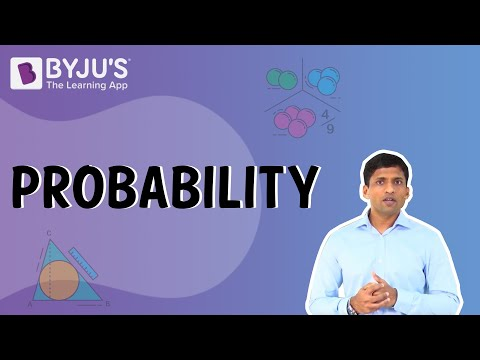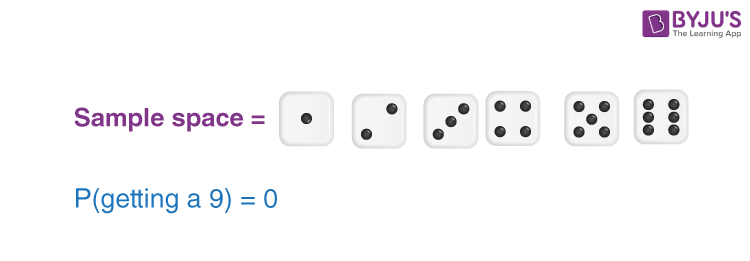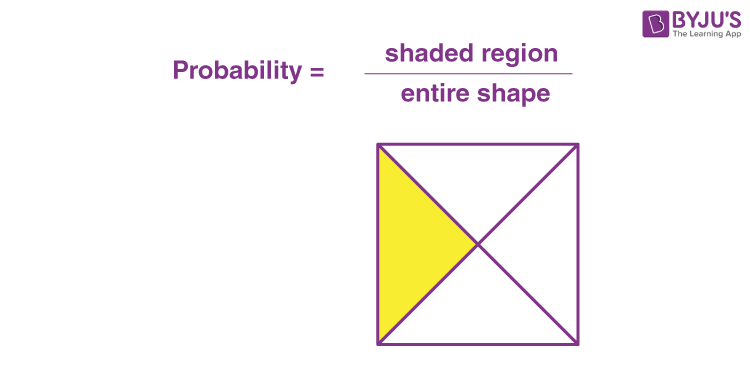# Probability Class 10 Notes Chapter 15

According to the CBSE Syllabus 2023-24, this chapter has been renumbered as Chapter 14.

## Class 10 Maths Chapter 15 Probability Notes

CBSE Class 10 Maths Chapter 15 Probability Notes are provided here in detail. In this article, we are going to learn the definition of probability, experimental probability, theoretical probability and the different terminologies used in probability with complete explanations.

Students can refer to the short notes and MCQ questions along with separate solution pdf of this chapter for quick revision from the links below:

## What Is Probability?

The branch of mathematics that measures the uncertainty of the occurrence of an event using numbers is called probability. The chance that an event will or will not occur is expressed on a scale ranging from 0-1.
It can also be represented as a percentage, where 0% denotes an impossible event and 100 % implies a certain event.
Probability of an Event E is represented by P(E).
For example, the probability of getting a head when a coin is tossed is equal to 1/2. Similarly, the probability of getting a tail when a coin is tossed is also equal to 1/2.
Hence, the total probability will be:
P(E) = 1/2 + 1/2 = 1
Know more about probability by clicking here.

### Event and outcome

An Outcome is a result of a random experiment. For example, when we roll a dice getting six is an outcome.
An Event is a set of outcomes. For example, when we roll dice, the probability of getting a number less than five is an event.
Note: An event can have a single outcome.

To know more about Types of Events, visit here.

## Experimental Probability

Experimental probability can be applied to any event associated with an experiment that is repeated a large number of times.
A trial is when the experiment is performed once. It is also known as empirical probability.
Experimental or empirical probability: P(E) =Number of trials  where the event occurred/Total Number of Trials
Example: In a day, a shopkeeper is able to sell 15 balls, out of which 6 were red balls. Find the probability of selling red balls on the next day of his sales.
Given, the total number of balls sold = 15
Number of red balls sold = 6
Probability of red balls = 6/15 = 2/5

To know more about Experimental Probability, visit here.

## Theoretical Probability

Theoretical Probability, P(E) = Number of Outcomes Favourable to E / Number of all possible outcomes of the experiment

Here we assume that the outcomes of the experiment are equally likely.
Example: Find the probability of picking up a red ball from a basket that contains 5 red and 7 blue balls.
Solution: Number of possible outcomes = Total number of balls = 5+7 = 12
Number of favourable outcomes = Number of red balls = 5
Hence,
Probability, P(red) = 5/12To know more about Theoretical Probability, visit here.

### Elementary Event

An event having only one outcome of the experiment is called an elementary event.
Example: Take the experiment of tossing a coin n number of times. One trial of this experiment has two possible outcomes: Heads(H) or Tails(T). So for an individual toss, it has only one outcome, i.e. Heads or Tails.

### Sum of Probabilities

The sum of the probabilities of all the elementary events of an experiment is one.
Example: take the coin-tossing experiment. P(Heads) + P(Tails )

(1/2)+ (1/2) =1

### Impossible Event

An event that has no chance of occurring is called an Impossible event, i.e. P(E) = 0.
E.g., The probability of getting a 7 on a roll of a die is 0. As 7 can never be an outcome of this trial.### Sure event

An event that has a 100% probability of occurrence is called a sure event. The probability of occurrence of a sure event is one.
E.g., What is the probability that a number obtained after throwing a die is less than 7?
So,  P(E) = P(Getting a number less than 7) = 6/6= 1

### Range of Probability of an event

Probability can range between 0 and 1, where 0 probability means the event to be an impossible one and probability of 1 indicates a certain event i.e. 0 ≤P (E) ≤ 1.

## Geometric Probability

Geometric probability is the calculation of the likelihood that one will hit a particular area of a figure. It is calculated by dividing the desired area by the total area. In the case of Geometrical probability, there are infinite outcomes.## Complementary Events

Complementary events are two outcomes of an event that are the only two possible outcomes. This is like flipping a coin and getting heads or tails.

$$\begin{array}{l}P(E)+P(\bar{E}) = 1\end{array}$$
$$\begin{array}{l}\text{where E and } \bar{E} \text{ are complementary events.}\end{array}$$
$$\begin{array}{l}\text{ The event } \bar{E} \text{ , representing ‘not E’, is called the complement of the event E.}\end{array}$$

The best example of complementary events is flipping a coin, where ‘getting a head’ complement the event of ‘getting a tail’.
To know more about Complementary Events, visit here.

## Probability for Class 10 Examples

Example 1:

A bag contains only lemon-flavoured candies. Arjun takes out one candy without looking into the bag. What is the probability that he takes out an orange-flavoured candy?

Solution:

Let us take the number of candies in the bag to be 100.

Number of orange-flavoured candies = 0 [since the bag contains only lemon-flavoured candies]

Hence, the probability that he takes out an orange-flavoured candy is:

P (Taking orange-flavoured candy) = Number of orange-flavoured candies / Total number of candies.

= 0/100 = 0

Hence, the probability that Arjun takes out an orange-flavoured candy is 0.

This proves that the probability of an impossible event is 0.

Example 2:

A game of chance consists of spinning an arrow that comes to rest, pointing at any one of the numbers such as 1, 2, 3, 4, 5, 6, 7, or 8, and these are equally likely outcomes. What is the probability that it will point at? (i)8 (ii) Number greater than 2 (iii) Odd numbers

Solution:

Sample Space = {1, 2, 3, 4, 5, 6, 7, 8}

Total Numbers = 8

(i) Probability that the arrow will point at 8:

Number of times we can get 8 = 1

P (Getting 8) = 1/8.

(ii) Probability that the arrow will point at a number greater than 2:

Number greater than 2 = 3, 4, 5, 6, 7, 8.

No. of numbers greater than 2 = 6

P (Getting numbers greater than 2) = 6/8 = 3/4.

(iii) Probability that the arrow will point at the odd numbers:

Odd number of outcomes = 1, 3, 5, 7

Number of odd numbers = 4.

P (Getting odd numbers) = 4/8 = ½.

### Related Articles:

Stay tuned with BYJU’S – The Learning App and download the app to learn all Maths-related concepts easily by exploring more videos.

Test your Knowledge on Probability Class 10